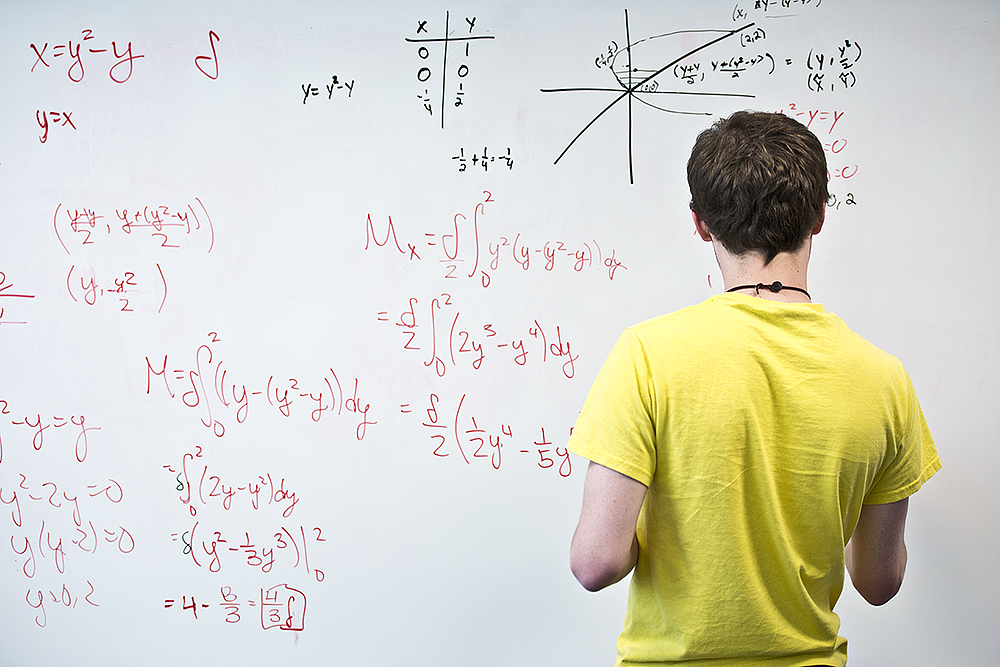# Here’s a list of math classes offered at KCC this fallInterested in taking a math class this fall but aren’t sure where to start? Below is a list of math classes offered this fall at Kellogg Community College, along with the relevant course descriptions.

The first Fall 2023 classes at Kellogg Community College start Aug. 28, and the semester ends Dec. 15. If you’re not a student yet but would like to sign up for fall classes, you can apply now for free online at kellogg.edu/apply. Current students can register for classes any time online via the Bruin Portal.

For help signing up, contact the KCC Admissions Office at 269-965-4153 or adm@kellogg.edu. For more details about the courses listed below, click here to view our Course Catalog, filter by “Fall Semester 2023” in the “Term” section under “Advanced Search,” then filter by subject.

## MATH 102: Practical Algebra (4 credits)

This course is designed for students pursuing a field of study outside of mathematics, science and engineering. Mathematical application will be an emphasis and real-life applications will be presented in disciplinary and career context when appropriate. Topics include: a review of operations with integers and rational numbers, ratios and proportions, percent, solutions of linear and quadratic equations, graphs of linear and exponential functions, linear and exponential models, intro to data and measures of central tendency.

## MATH 105: Beginning Algebra (4 credits)

Course content includes operations on integers and rational numbers; geometric formulas; algebraic expressions; solutions of linear equations and inequalities; graphs of linear equations and linear systems; systems of linear equations in two variables; polynomials and factoring; rational expressions and equations; and radical expressions and equations.

## MATH 105C: Beginning Algebra Lab (1 credit)

This course must be taken concurrently with MATH 105. Students in this course will develop the foundational skills needed to be successful in Beginning Algebra. Academic support for student success provided.

## MATH 111: Mathematics for Elementary Teachers 1 (4 credits)

This course is designed for students majoring in elementary education and to give mathematical understandings and skills necessary to teach in elementary schools. Logical developments and structure are emphasized throughout. Topics included are sets, natural numbers, integers, rational numbers, irrational numbers, numeration systems, calculator applications and selected topics from number theory. Students are recommended to have a scientific calculator. Specifications will be discussed by the instructor.

## MATH 115: Math for Liberal Arts (4 credits)

This is a liberal arts mathematics course designed primarily for students whose programs of study have no further mathematics requirements. Its purpose is to develop an awareness of the use of mathematics in the world around us. Emphasis will be on the communication of mathematical ideas, problem solving, applications and the historical nature of mathematics. Specific topics for this course include: logic and reasoning, mathematics of finance and investment, probability, statistics, graph theory and geometry.

## MATH 115C: Math for Liberal Arts Lab (1 credit)

Students in this course will develop foundational and auxiliary skills to support a successful outcome in MATH 115.

## MATH 125: College Algebra (4 credits)

Topics included are polynomials, fundamental theorem of algebra, relations and functions including rational functions, logarithmic functions, exponential functions, inverse functions including graphing and applications of each relation and function.

## MATH 125C: College Algebra Lab (1 credit)

This course must be taken concurrently with MATH 125. Content to reinforce topics in Math 125 will be emphasized; skills for developing mathematics proficiency will be mastered.

## MATH 130: Statistics (3 credits)

A study of basic descriptive statistics, introduction to probability, probability distributions, sampling theory, hypothesis testing, analysis distributions, sampling theory, hypothesis testing, analysis of variance, and linear correlation and regression. Students are required to have a graphing calculator. Specifications will be made by the instructor.

## MATH 130C: Statistics Lab (1 credit)

This course must be taken concurrently with MATH 130. Students in this course will develop foundational and auxiliary skills to support a successful outcome in MATH 130. It is highly recommended for students who are not familiar with the Texas Instruments family of calculators or are shy around technology. We will be incorporating proper college studying techniques and in-person attendance is required and graded. Completion of work in their section of MATH 130 will be a significant factor in this course.

## MATH 132: Trigonometry (3 credits)

This course is a study of trigonometric functions, their inverses and graphs, identities, equations, radian measure and solution of triangles. Students are required to have a graphing calculator. Specifications will be made by the instructor.

## MATH 140: Preparation for Calculus (4 credits)

Topics in this course include: introductory plane geometry, algebraic functions and their graphs, introduction to theory of equations, combinations and binomial theorem, exponential and logarithmic functions, trigonometric functions and arithmetic and geometric sequences. Students are required to have a graphing calculator. Specifications will be made by the instructor.

## MATH 141: Calculus 1 (5 credits)

Topics in this course include: limits, differentiation of algebraic and transcendental functions, the definite integral, fundamental theorem of calculus, and applications. Students are required to have a graphing calculator. Specifications will be made by the instructor.

## MATH 241: Calculus 3 (4 credits)

Vector calculus, partial derivatives, multiple integrals and applications.

Math instruction is an integral part of KCC’s Associate in Science transfer degree for STEM majors. For more information, visit kellogg.edu/experience. For more details about signing up for classes at KCC, visit kellogg.edu/registration.